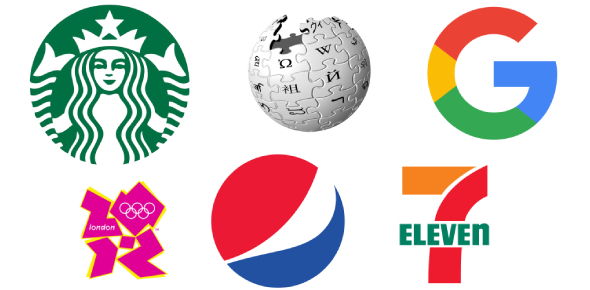# Sacientific Concepts Test 4a

24 Questions | Total Attempts: 138Settings• 1.
A science teacher asked her students to make a chart identifying the physical properties of each object below:Which of the following would be best to use for this char of physical properties?
• A.

A- age, color, length, mass ion 1

• B.

B- hardness, mass, name, odor 2

• C.

C- color, hardness, mass, length

• 2.
The characteristic of an object that has mass and takes up space is:
• A.

A- volume

• B.

B- mass

• C.

C- weight

• 3.
One of the illustrations below is used to measure the amount of matter that      makes up an object is:
• A.

Option 1

• B.

Option 2

• C.

Option 3

• 4.
The parts of an electromagnet are:
• A.

A- battery, battery holder, nail, wire

• B.

B- battery, battery holder, switch, wire

• C.

C- battery, battery holder, nail, bulb

• 5.
The two phases of matter in the next illustration are:
• A.

A- solid and liquid

• B.

B-solid and gas

• C.

C- liquid and gas

• 6.
What is electricity transformed into when you turn on a TV?
• A.

A- light, motion, and sound

• B.

B- light, sound, and heat

• C.

C- light, heat, and motion

• 7.
Property that compares an object’s mass to its volume is called:
• A.

A-matter

• B.

B-property

• C.

C- density

• 8.
Which of the following colors represents the greatest density in the illustration below:
• A.

A- red

• B.

B-green

• C.

C-blue

• 9.
One of the following is a property of solid particles:
• A.

A-tightly packed and held in place

• B.

B-far apart

• C.

C-close to each other but they are not held tightly in place

• 10.
Which of the following will be attracted to the magnet:
• A.

Eraser

• B.

Paper clips

• C.

Pencil

• 11.
The  evaporation process in  water  cycle        chart is number:
• A.

A

• B.

B

• C.

• 12.
The illustration that represents the liquid state of matter is:
• A.

Option 1

• B.

Option 2

• C.

Option 3

• 13.
“Boiling egg” is a process that changes the egg from liquid to solid. This            change is :
• A.

A-melting

• B.

B- chemical change

• C.

C- physical change

• 14.
An electric charge in motion is called:
• A.

A- electric current

• B.

B- insulator

• C.

C-conductor

• 15.
Which one of the following circuits shows a complete circuit?
• A.

Option 1

• B.

Option 2

• C.

Option 3

• 16.
Look carefully at the illustration below. What form of energy is created when a magnet moves near a wire?
• A.

A-kinetic energy

• B.

B-light energy

• C.

C-electrical energy

• 17.
In the illustration below the number that points to the resistor is:
• A.

A- 1

• B.

B-2

• C.

C- 3

• 18.
Series circuit is a type of circuits that:
• A.

A- the current moves through it when there is a broken loop

• B.

B- has two or more paths through which electric charges may flow

• C.

C- electric charge can flow only in one circular path

• 19.
All magnets have two:
• A.

A- sides

• B.

B- names

• C.

C- poles

• 20.
Which letter of the following magnets represents “repel”:
• A.

A

• B.

B

• C.

C

• 21.
In the next illustration, if lamp 1 is removed from its holder, what will        happen to lamp 2 :
• A.

A- it will stay working

• B.

B- it will not work

• C.

C-it will get dimmer

• 22.
The best symbol  below that represents the resistor is:
• A.

Option 1

• B.

Option 2

• C.

Option 3

• 23.
Ahmad needs to buy a light bulb which saves energy for his room. He found       two types of them, the incandescent light bulb and the fluorescent light bulb.
• A.

A- fluorescent

• B.

B- incandescent

• C.

C- electronic

• 24.
The circuit below is one type of circuits that are used at homes and schools.         is :
• A.

A- new circuit

• B.

B- series circuit

• C.

C- parallel circuitBack to top